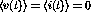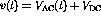Next: AC Circuit Elements Up: PHYSICS LECTURE NOTES PHYS Previous: Problems

# Alternating Current Circuits

We now consider circuits where the currents and voltages may vary with time (V=V(t), I=I(t) (also Q=Q(t))). These lectures will concentrate on the special case in which the signals are periodic, with time average values of zero (). Circuits with these signals are referred to as alternating current (AC) circuits. In general signals will have both DC and AC properties (). We will concentrate only on the AC components and assume that the DC properties can be treated separately using the methods of the previous lectures.

The algebraic equations representing Kirchoff's laws for DC circuits will take the form of differential equations for AC circuits. So now is a good time to review your differential equations and complex number theory because we will use it.

Doug Gingrich
Tue Jul 13 16:55:15 EDT 1999